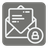Caesar Cipher (encryptor)
Elementary+
English RU

This mission is the part of the set. Another one - Caesar cipher decriptor.

Your mission is to encrypt a secret message (text only, without special chars like "!", "&", "?" etc.) using Caesar cipher where each letter of input text is replaced by another that stands at a fixed distance. For example ("a b c", 3) == "d e f"Input: A secret message as a string (lowercase letters only and white spaces)

Output: The same string, but encrypted

Example:

```to_encrypt("a b c", 3) == "d e f"
to_encrypt("a b c", -3) == "x y z"
to_encrypt("simple text", 16) == "iycfbu junj"
to_encrypt("important text", 10) == "swzybdkxd dohd"
to_encrypt("state secret", -13) == "fgngr frperg"
```

How it is used: For cryptography and to save important information.

Precondition:
0 < len(text) < 50
-26 < delta < 26